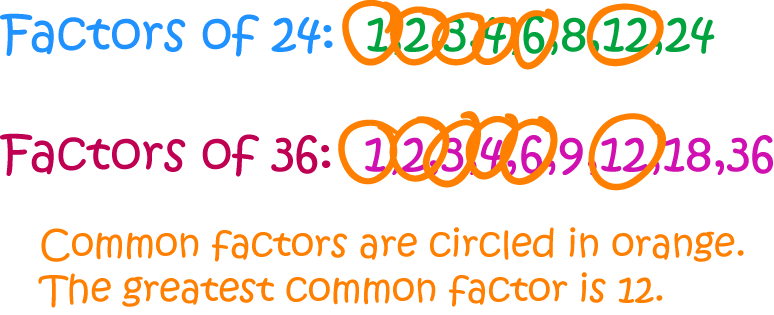Pull Down & Release to Refresh

# Definition of Greatest Common FactorThe greatest common factor of two numbers is the largest number that divides evenly into both numbers.

To find it, we write down all the factors of both numbers, and choose the largest number out of these that is a factor of both numbers.

It is abbreviated as "GCF".

Other names for the Greatest Common Factor are the "Greatest Common Divisor" and the "Highest Common Factor".

For example, the GCF of $24$ and $36$ is $12$ because both numbers have common factors of $1$, $2$, $3$, $4$, $6$ and $12$, and $12$ is the largest of these.

### Description

The aim of this dictionary is to provide definitions to common mathematical terms. Students learn a new math skill every week at school, sometimes just before they start a new skill, if they want to look at what a specific term means, this is where this dictionary will become handy and a go-to guide for a student.

### Audience

Year 1 to Year 12 students

### Learning Objectives

Learn common math terms starting with letter G

Author: Subject Coach
You must be logged in as Student to ask a Question.